### Construction of Q-Q and P-P Plots

If a normal probability-probability or quantile-quantile plot for the variable x is requested, the n nonmissing values of x are first ordered from smallest to largest: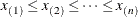If a Q-Q plot is requested (with a PLOT statement of the form PLOT yvariable*NQQ.), the ith-ordered value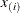is represented by a point with y-coordinateand x-coordinate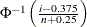, where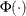is the standard normal distribution.

If a P-P plot is requested (with a PLOT statement of the form PLOT yvariable*NPP.), the ith-ordered valueis represented by a point with y-coordinateand x-coordinate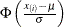, whereis the mean of the nonmissing x-values andis the standard deviation. If an x-value has multiplicity k (that is,), then only the point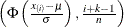is displayed.